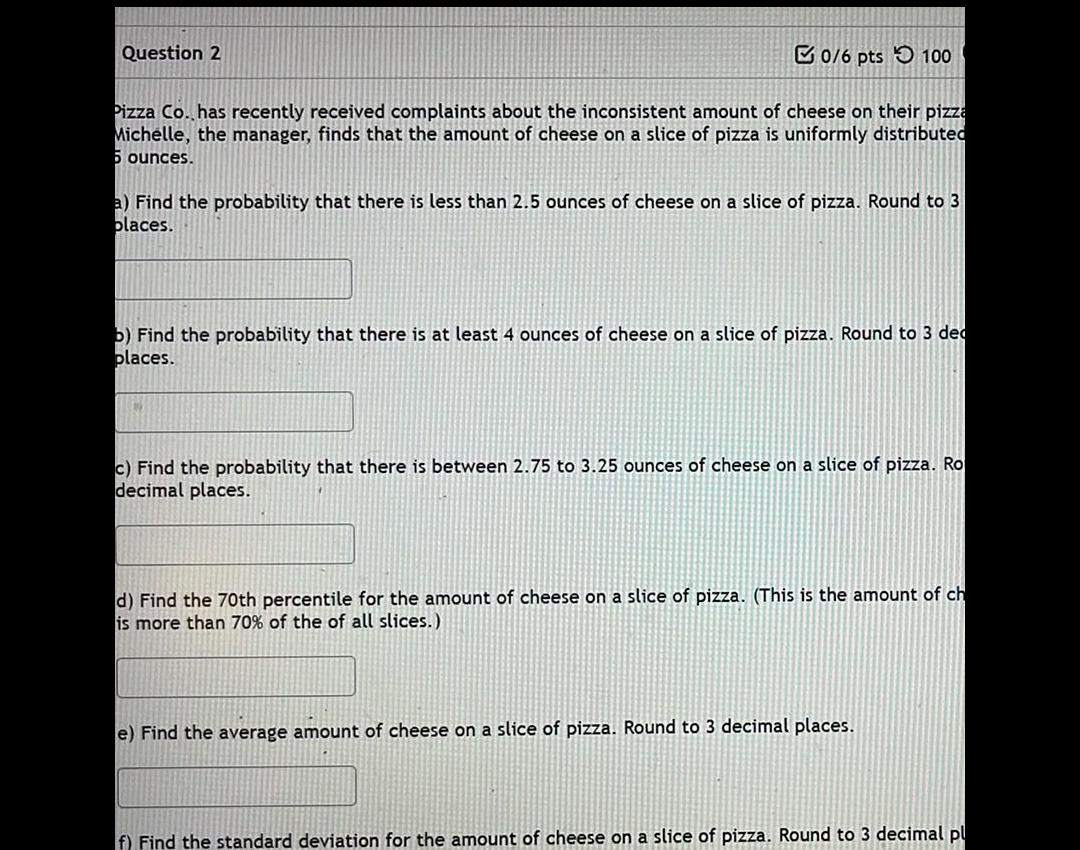Question:

# Question 2 0 6 pts 100 Pizza Co has recently received

Last updated: 3/20/2023Question 2 0 6 pts 100 Pizza Co has recently received complaints about the inconsistent amount of cheese on their pizza Michelle the manager finds that the amount of cheese on a slice of pizza is uniformly distributed 6 ounces a Find the probability that there is less than 2 5 ounces of cheese on a slice of pizza Round to 3 places b Find the probability that there is at least 4 ounces of cheese on a slice of pizza Round to 3 dec places c Find the probability that there is between 2 75 to 3 25 ounces of cheese on a slice of pizza Ro decimal places d Find the 70th percentile for the amount of cheese on a slice of pizza This is the amount of ch is more than 70 of the of all slices e Find the average amount of cheese on a slice of pizza Round to 3 decimal places f Find the standard deviation for the amount of cheese on a slice of pizza Round to 3 decimal pl